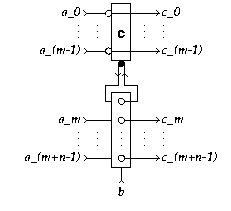EDIS: Guide | FAQ | New | Search | Bibliography | Index | Feedback

# k-Latch

## Specifications

### Informal

A k-Latch (k>0) has k+1 input terminals a_i (0<=i<k) and b, and k output terminals c_i (0<=i<k). It waits for a signal on exactly one of the a inputs and a signal on the b input. In contrast to a k-Sequencer, the environment must guarantee mutual exclusion of the a-inputs. Having received input signals on a_i (0<=i<k) and b, it produces a signal on output terminal c_i.

Rephrasing: Both the a and the c wire pair can be viewed as encoding a value by a One-Hot code. When the value is sent to the k-Latch via an a input, it stores (latches) this value until b is received. Reception of b reproduces the value via the corresponding c ouput.

### XDI

Schematic diagram for a k-Latch:[Zoom|FIG]

We provide the XDI specification for the a 3-Latch:

Specification in XDI model.

(Not available for general k.)

### Verdect

Parameterized definitions are not possible in VERDECT, but this sketch gives the general idea:

Specification in Verdect:

```
define L( a0?, ..., a(k-1)?, b?, c0!, ..., c(k-1)! ) =
pref *[ (a0? || b?); c0!
| ...
| (a(k-1)? || b?); c(k-1)!
]
end
```

The specification of the 3-Latch is as follows:

Specification in Verdect:

```define L( a0?, a1?, a2?, b?, c0!, c1!, c2! ) =
pref *[ (a0? || b?); c0!
| (a1? || b?); c1!
| (a2? || b?); c2!
]
end
```

.

### DI Algebra

Parameterized definitions are not possible in DI Algebra, but this sketch gives the general idea:

Specification in DI Algebra:

```I = { a0?, .. , a(k-1)?, b? }
O = { c0?, .. , c(k-1)! }

L(k,k) = [ (,i: 0<=i < k: ai? -> L(i,k),
b?      -> M(k,k)
]
L(i,k) = [ b? -> M(i,k), else -> CHAOS ], for 0<=i M(i,k), b? -> CHAOS]
M(i,k) = ci!;L(k,k)
```

We provide the specification for the 3-Latch:

Specification in DI Algebra.

## Properties

The XDI Report for the 3-Latch.

The roles of subscripts i can be permuted (on a_i and c_i simultaneously).

The k-Latch satisfies Rules Y' and Z^out, but not Z^inp (choice between a inputs).

## Implementations

### DI Decompositions

1. A 1-Latch is a Join.
2. A 2-Latch is a Latch.
3. A k-Latch is implemented by a Sequencer (not equ).
4. A (m+n)-Latch can be implemented with a k-nr-mixer and a (n+1)-Latch (equ):[Zoom|FIG]

### Using Boolean Gates

No information available

### Using Transistors

No information available

## Generalizations

The k-Latch can be generalized to an mxn-Decision-Wait, which awaits two choices, one between m inputs and the other between n inputs, and reports the combination as a choice among mn outputs. A k-Latch is a kx1-Decision-Wait.

## Miscellaneous

In terms of [Ebergen89, p. 88], the k-Latch is a CAL component.

The k-Latch is sometimes also referred to as Decision-Wait ([Lucassen94]) or kx1-Join.

## References

[Ebergen89, p. 88]
[Lucassen94]